# Percent Composition Empirical Molecular Formulas Molecular Formulas The

• Slides: 28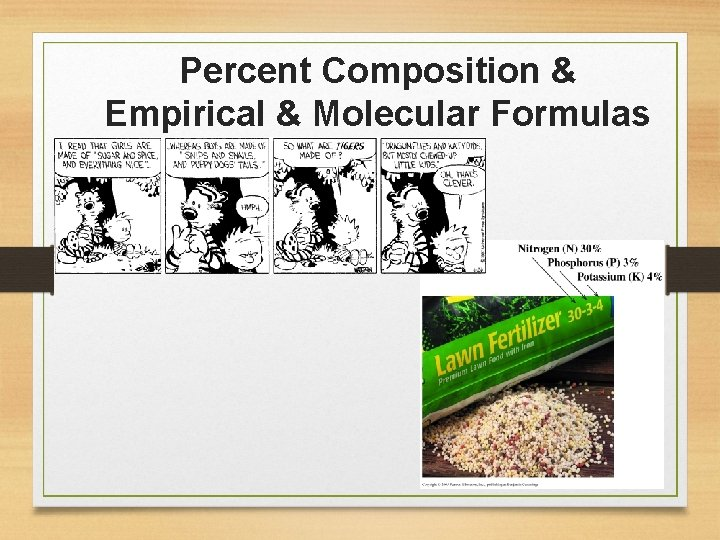Percent Composition & Empirical & Molecular Formulas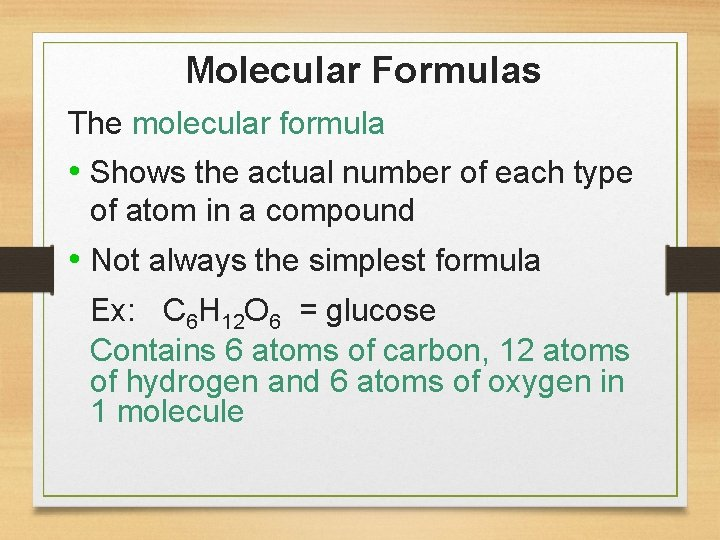Molecular Formulas The molecular formula • Shows the actual number of each type of atom in a compound • Not always the simplest formula Ex: C 6 H 12 O 6 = glucose Contains 6 atoms of carbon, 12 atoms of hydrogen and 6 atoms of oxygen in 1 molecule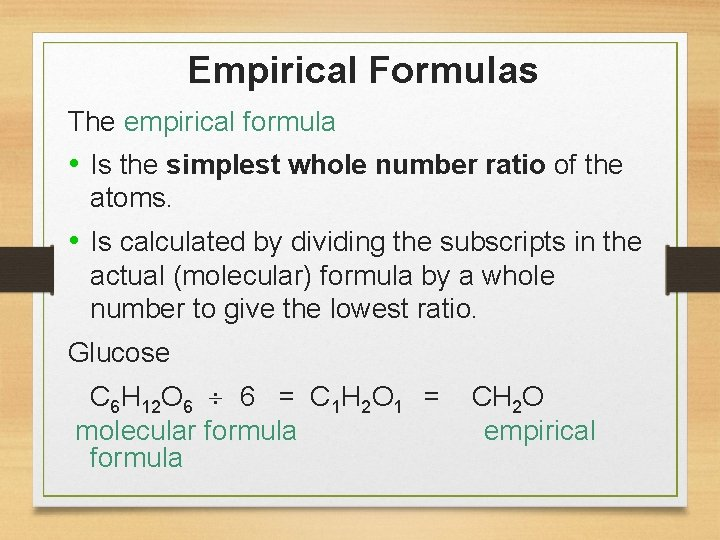Empirical Formulas The empirical formula • Is the simplest whole number ratio of the atoms. • Is calculated by dividing the subscripts in the actual (molecular) formula by a whole number to give the lowest ratio. Glucose C 6 H 12 O 6 6 = C 1 H 2 O 1 = CH 2 O molecular formula empirical formulaSome Molecular and Empirical Formulas • The molecular formula is the same or a multiple of the empirical. 4You Try… 1. What is the empirical formula for C 4 H 8? 2. What is the empirical formula for C 8 H 14? 3. Which is a possible molecular formula for CH 2 O? a) C 4 H 4 O 4 b) C 2 H 4 O 2 c) C 3 H 6 O 3 4. A compound has an empirical formula SN. If there are 4 atoms of N in one molecule, what is the molecular formula? Explain.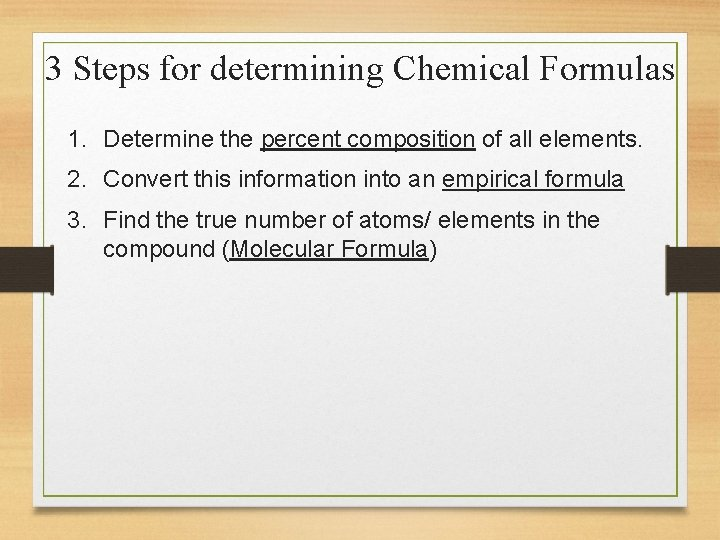3 Steps for determining Chemical Formulas 1. Determine the percent composition of all elements. 2. Convert this information into an empirical formula 3. Find the true number of atoms/ elements in the compound (Molecular Formula)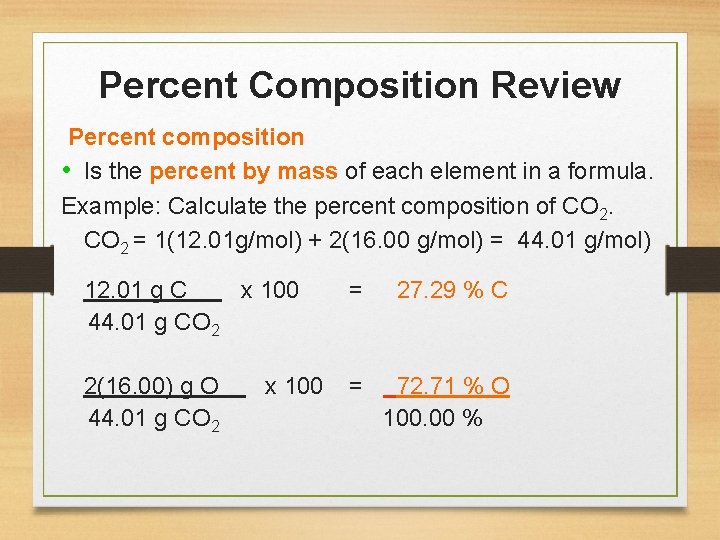Percent Composition Review Percent composition • Is the percent by mass of each element in a formula. Example: Calculate the percent composition of CO 2 = 1(12. 01 g/mol) + 2(16. 00 g/mol) = 44. 01 g/mol) 12. 01 g C x 100 = 27. 29 % C 44. 01 g CO 2 2(16. 00) g O x 100 = 72. 71 % O 44. 01 g CO 2 100. 00 %You Try… 5. What is the percent composition of lactic acid, C 3 H 6 O 3, a compound that appears in the blood after vigorous activity? 6. The chemical isoamyl acetate C 7 H 14 O 2 gives the odor of pears. What is the percent carbon in isoamyl acetate?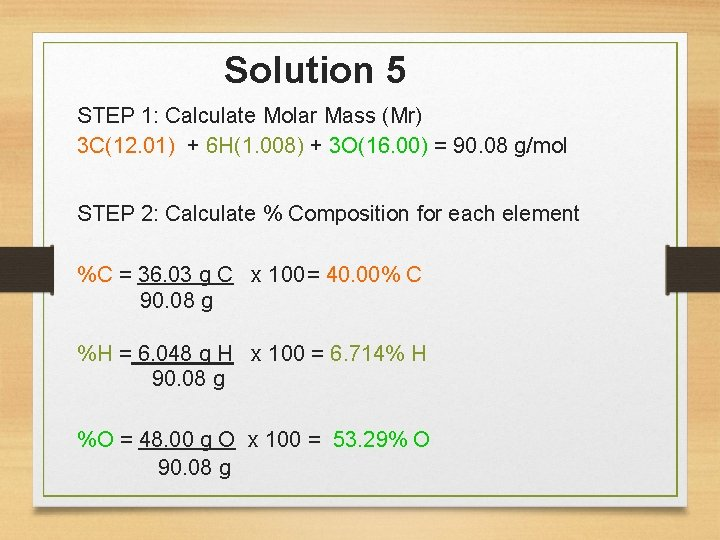Solution 5 STEP 1: Calculate Molar Mass (Mr) 3 C(12. 01) + 6 H(1. 008) + 3 O(16. 00) = 90. 08 g/mol STEP 2: Calculate % Composition for each element %C = 36. 03 g C x 100= 40. 00% C 90. 08 g %H = 6. 048 g H x 100 = 6. 714% H 90. 08 g %O = 48. 00 g O x 100 = 53. 29% O 90. 08 g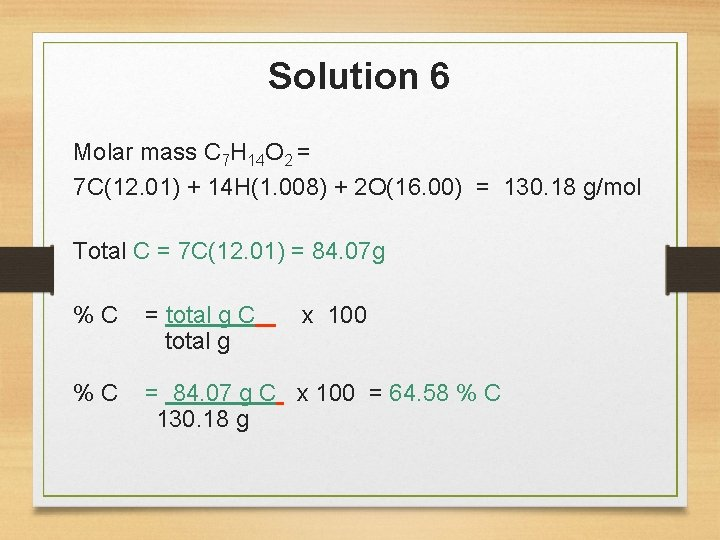Solution 6 Molar mass C 7 H 14 O 2 = 7 C(12. 01) + 14 H(1. 008) + 2 O(16. 00) = 130. 18 g/mol Total C = 7 C(12. 01) = 84. 07 g % C = total g C x 100 total g % C = 84. 07 g C x 100 = 64. 58 % C 130. 18 g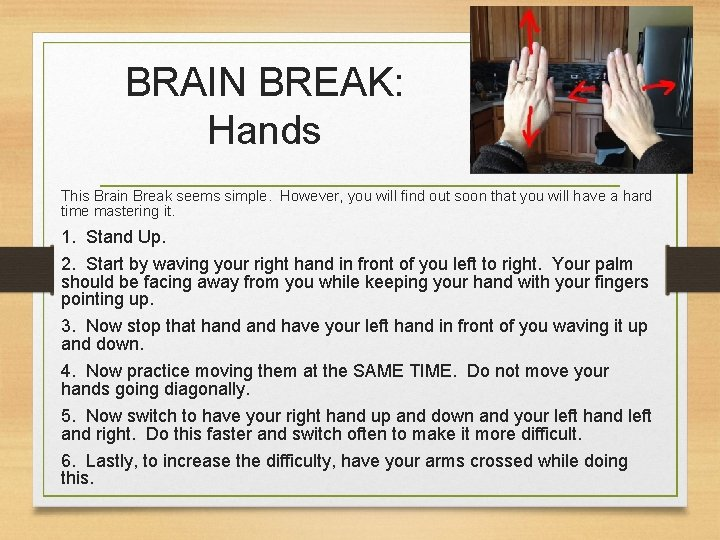BRAIN BREAK: Hands This Brain Break seems simple. However, you will find out soon that you will have a hard time mastering it. 1. Stand Up. 2. Start by waving your right hand in front of you left to right. Your palm should be facing away from you while keeping your hand with your fingers pointing up. 3. Now stop that hand have your left hand in front of you waving it up and down. 4. Now practice moving them at the SAME TIME. Do not move your hands going diagonally. 5. Now switch to have your right hand up and down and your left hand left and right. Do this faster and switch often to make it more difficult. 6. Lastly, to increase the difficulty, have your arms crossed while doing this.Calculating Empirical Formula 1. If given % composition, assume 100 g of sample 2. Convert mass of each element to moles (g mol) 3. Divide each of these numbers by the smallest number 4. If necessary, multiply by the smallest number possible to make each a whole number 5. These whole numbers are the subscripts in the empirical formula called mole ratioThe percentage composition of a compound is found to be 32. 4% sodium, 22. 5% sulfur, 45. 1 % oxygen. Determine the empirical formula. Step 1: Assume 100 g of Sample 32. 4% Na 32. 4 g Na 22. 5% S 22. 5 g S 45. 1% O 45. 1 g O Step 2: Convert grams to moles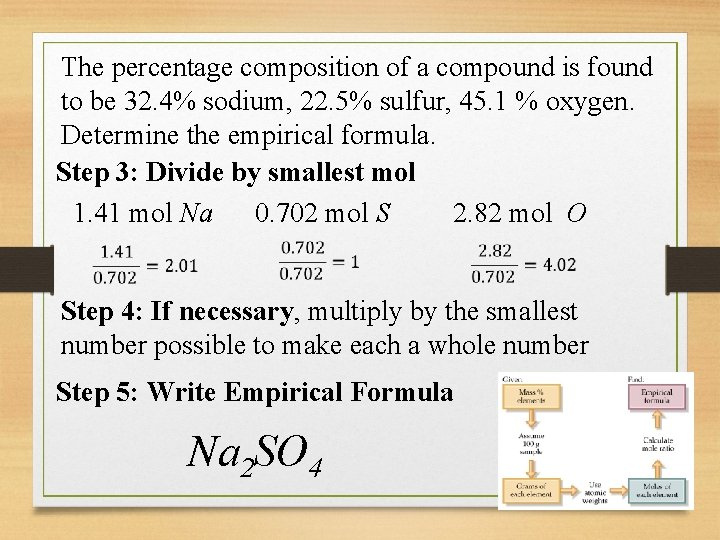The percentage composition of a compound is found to be 32. 4% sodium, 22. 5% sulfur, 45. 1 % oxygen. Determine the empirical formula. Step 3: Divide by smallest mol 1. 41 mol Na 0. 702 mol S 2. 82 mol O Step 4: If necessary, multiply by the smallest number possible to make each a whole number Step 5: Write Empirical Formula Na 2 SO 4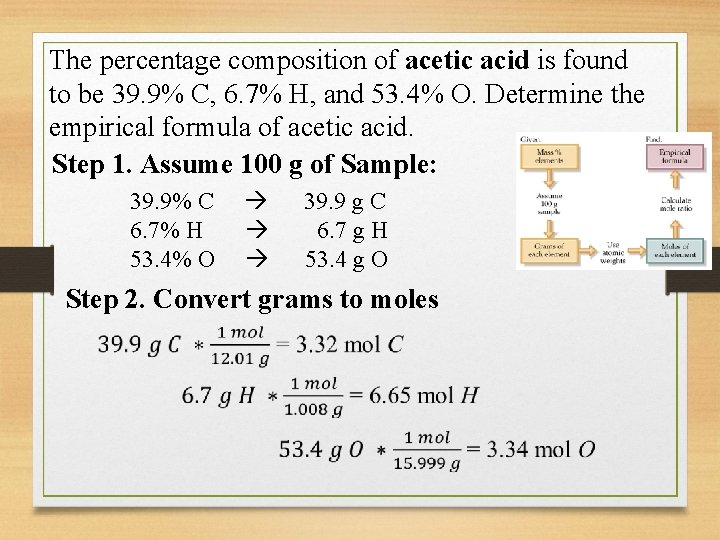The percentage composition of acetic acid is found to be 39. 9% C, 6. 7% H, and 53. 4% O. Determine the empirical formula of acetic acid. Step 1. Assume 100 g of Sample: 39. 9% C 6. 7% H 53. 4% O 39. 9 g C 6. 7 g H 53. 4 g O Step 2. Convert grams to moles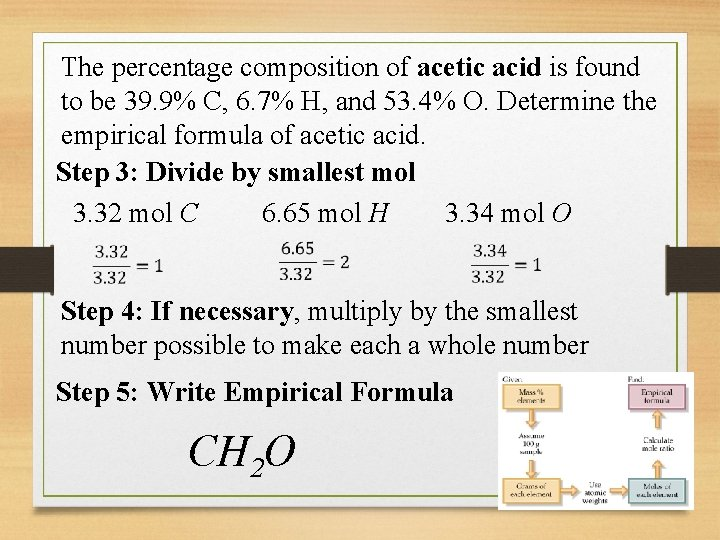The percentage composition of acetic acid is found to be 39. 9% C, 6. 7% H, and 53. 4% O. Determine the empirical formula of acetic acid. Step 3: Divide by smallest mol 3. 32 mol C 6. 65 mol H 3. 34 mol O Step 4: If necessary, multiply by the smallest number possible to make each a whole number Step 5: Write Empirical Formula CH 2 O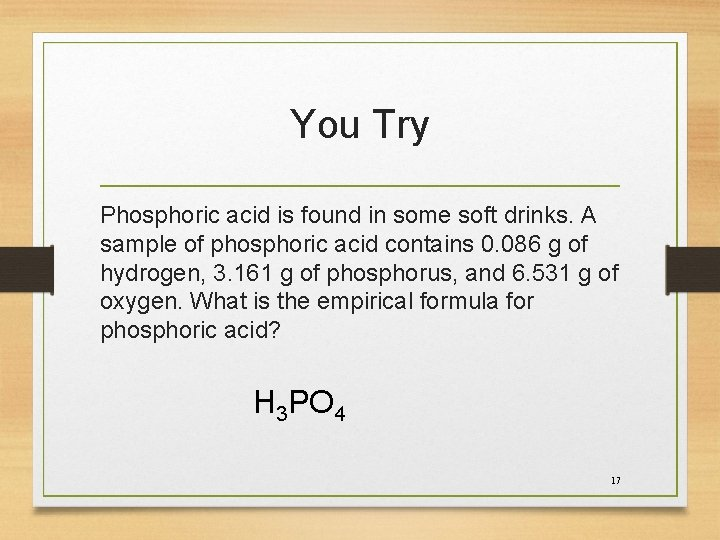You Try Phosphoric acid is found in some soft drinks. A sample of phosphoric acid contains 0. 086 g of hydrogen, 3. 161 g of phosphorus, and 6. 531 g of oxygen. What is the empirical formula for phosphoric acid? H 3 PO 4 17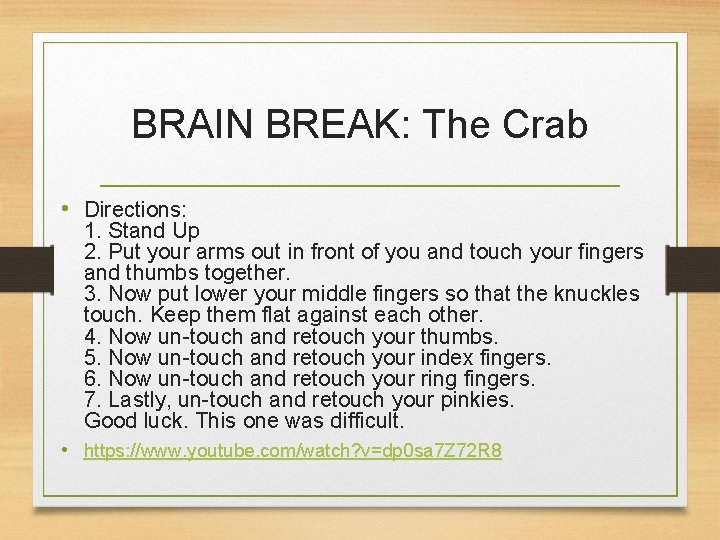BRAIN BREAK: The Crab • Directions: 1. Stand Up 2. Put your arms out in front of you and touch your fingers and thumbs together. 3. Now put lower your middle fingers so that the knuckles touch. Keep them flat against each other. 4. Now un-touch and retouch your thumbs. 5. Now un-touch and retouch your index fingers. 6. Now un-touch and retouch your ring fingers. 7. Lastly, un-touch and retouch your pinkies. Good luck. This one was difficult. • https: //www. youtube. com/watch? v=dp 0 sa 7 Z 72 R 8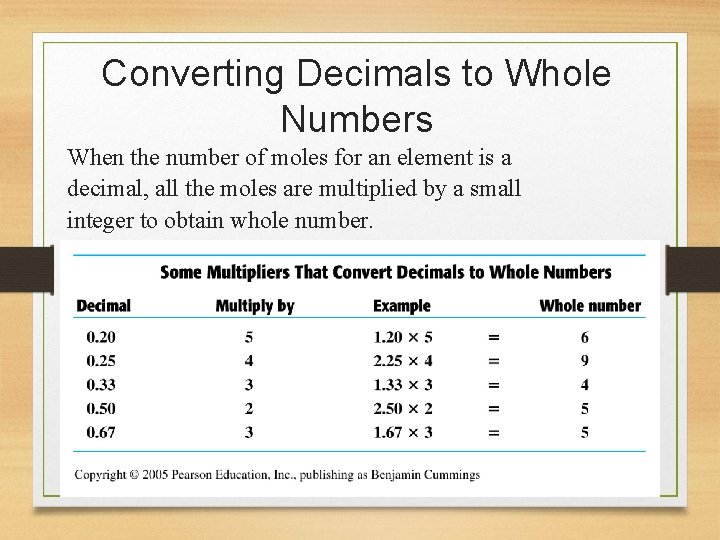Converting Decimals to Whole Numbers When the number of moles for an element is a decimal, all the moles are multiplied by a small integer to obtain whole number.Aspirin is 60. 0% C, 4. 5 % H and 35. 5 % O. Calculate its empirical formula. Step 1. Assume 100 g of Sample: 60. 0% C 4. 5% H 35. 5% O 60. 0 g C 4. 5 g H 35. 5 g O Step 2. Convert grams to molesAspirin is 60. 0% C, 4. 5 % H and 35. 5 % O. Calculate its empirical formula. Step 3: Divide by smallest mol 5. 00 mol C 4. 46 mol H 2. 22 mol O Step 4: If necessary, multiply by the smallest number possible to make each a whole number Step 5: Write Empirical Formula C 9 H 8 O 4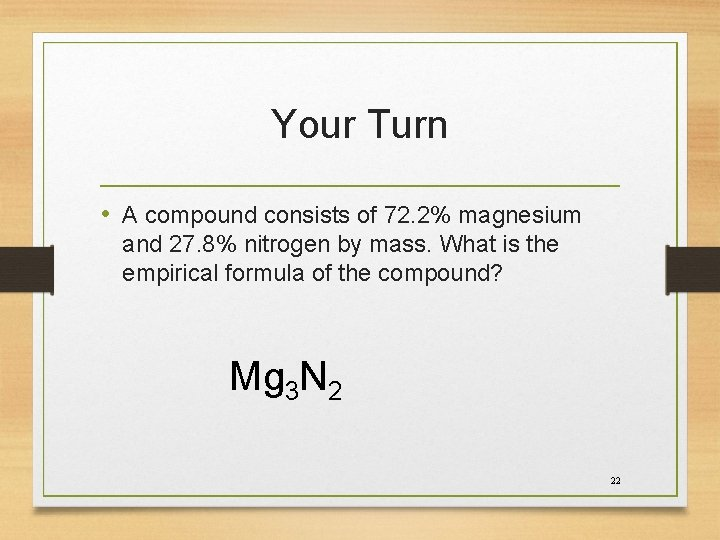Your Turn • A compound consists of 72. 2% magnesium and 27. 8% nitrogen by mass. What is the empirical formula of the compound? Mg 3 N 2 22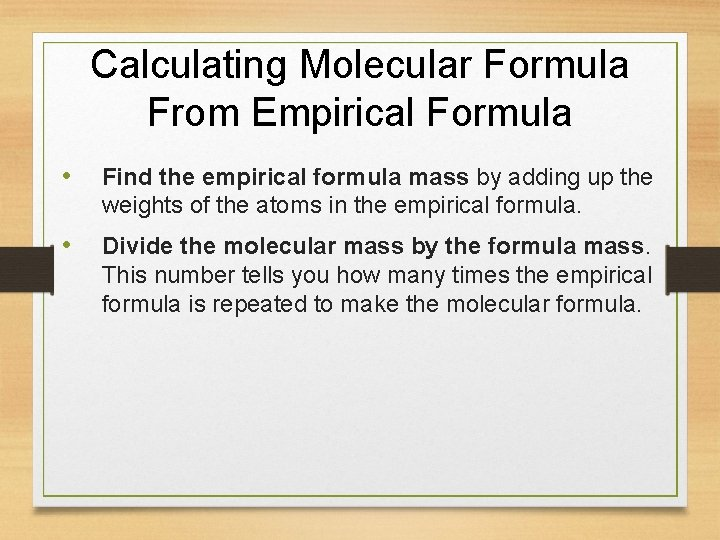Calculating Molecular Formula From Empirical Formula • Find the empirical formula mass by adding up the weights of the atoms in the empirical formula. • Divide the molecular mass by the formula mass. This number tells you how many times the empirical formula is repeated to make the molecular formula.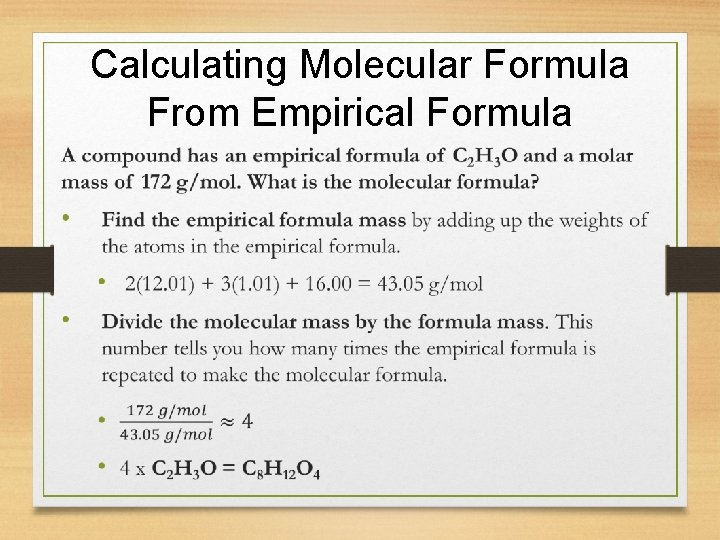Calculating Molecular Formula From Empirical Formula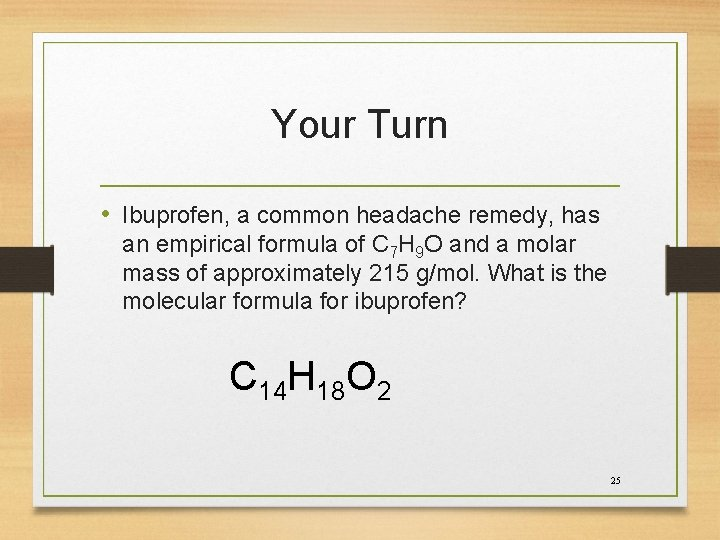Your Turn • Ibuprofen, a common headache remedy, has an empirical formula of C 7 H 9 O and a molar mass of approximately 215 g/mol. What is the molecular formula for ibuprofen? C 14 H 18 O 2 25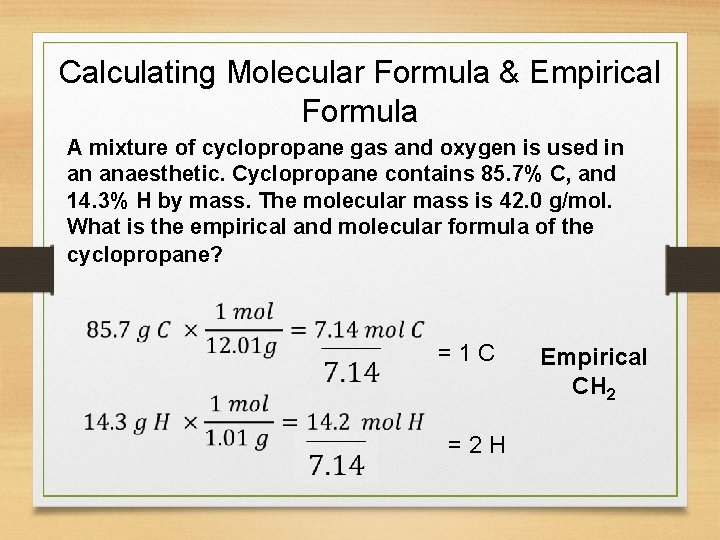Calculating Molecular Formula & Empirical Formula A mixture of cyclopropane gas and oxygen is used in an anaesthetic. Cyclopropane contains 85. 7% C, and 14. 3% H by mass. The molecular mass is 42. 0 g/mol. What is the empirical and molecular formula of the cyclopropane? = 1 C = 2 H Empirical CH 2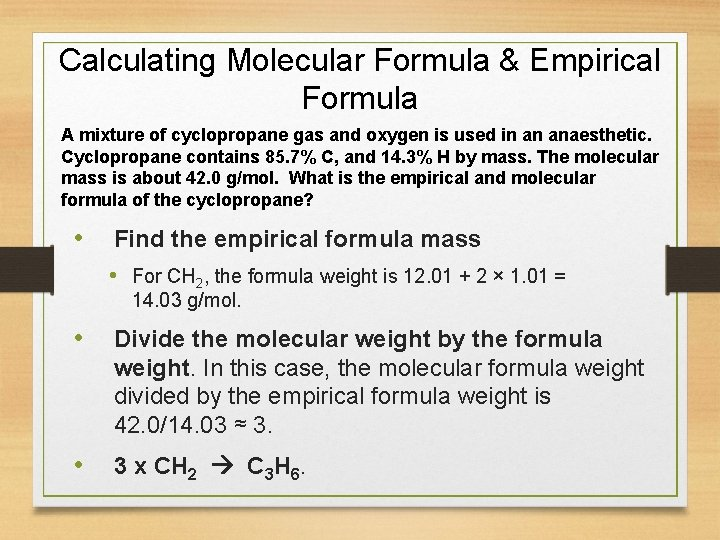Calculating Molecular Formula & Empirical Formula A mixture of cyclopropane gas and oxygen is used in an anaesthetic. Cyclopropane contains 85. 7% C, and 14. 3% H by mass. The molecular mass is about 42. 0 g/mol. What is the empirical and molecular formula of the cyclopropane? • Find the empirical formula mass • For CH 2, the formula weight is 12. 01 + 2 × 1. 01 = 14. 03 g/mol. • Divide the molecular weight by the formula weight. In this case, the molecular formula weight divided by the empirical formula weight is 42. 0/14. 03 ≈ 3. • 3 x CH 2 C 3 H 6.YOUR TURN Epinephrine (adrenaline) is a hormone secreted into the bloodstream in times of danger and stress. It is 59. 0% carbon, 7. 1% hydrogen, 6. 2% oxygen, and 7. 7% nitrogen by mass. Its molar mass is about 180 g/mol. Find the empirical and molecular formulas of epinephrine. Empirical C 9 H 13 O 3 N Molecular C 9 H 13 O 3 N 28